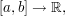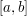• 11 years 20 weeks ago Random sampling using Markov chains

It's because there have been no revisions to the article. Perhaps we should display the tab regardless, to avoid confusion.

• 11 years 20 weeks ago Different kinds of Tricki article

I agree, and this dates from when I used to write articles differently. I'll try to do something about it soon.

• Sune Kristian Jakbsen (not verified) 11 years 20 weeks ago Random sampling using Markov chains

• Jonah (not verified) 11 years 20 weeks ago Elementary examples of invariants

Though it doesn't really belong in this article, there's a very clever proof of the fourth example (and many similar problems) which should certainly go somewhere in the Tricki. I believe it's called the garden-path argument, though I can't find who invented it.

Given such a tiling of R, create a graph G whose vertices are the corners of the tiles and whose edges connect the corners along the sides of integer length. For tiles with four sides of integer length, just include two parallel sides (it doesn't matter which) as edges in G rather than all four. Now the degree of each vertex is the number of tiles for which it's a corner. It's easy to see that this is 1 for the four corners of R, and 2 or 4 for all other vertices.

If we arbitrarily make a path starting at one of these four corners which repeats no edges, we can only get stuck at another corner, since all other vertices have even degree. So there exists a path between two distinct corners of R which only moves parallel to the sides of R in integral steps, and so at least one side of R has integral length.

One can also use this to prove, for instance, that an m-by-n rectangle can be tiled with 1-by-k polyominoes if and only if k divides m or n.

• Sune Kristian Jakobsen (not verified) 11 years 20 weeks ago Different kinds of Tricki article

• 11 years 20 weeks ago

The remark stems from a previous version of the article, in which I had included Lebesgue's proof of the Weierstrass approximation theorem as an example of the technique, and I think the following is what I had in mind.

Define a relation ~ on collections of functionssaying that A ~ B if and only if any function in A can be uniformly approximated arbitrarily well by a function in B. Weierstrass approximation is the statement that if

• X is the collection of continuous functions onand
• Y is the collection of polynomials on,

then X ~ Y. Lebesgue's proof proceeds via the intermediate collection Z, the collection of piecewise linear functions on. Now, ~ is not a symmetric relation in general, which is why I made that remark.

That said, I guess ~ is an equivalence relation if one restricts it to just the collections X, Y and Z, though one does not really need to know this. (One only needs the transitivity, which follows from the unrestricted version of the relation.)

Actually, having re-read the quick description of the article, I now see why this is an issue: I wrote "show that they are both 'equivalent' to a third", whereas I was thinking "show that X ~ Z and Z ~ Y for some Z".

So either the quick description should be changed, or that remark should go. I'm not sure if there are interesting examples where ~ is not be an equivalence relation restricted to X, Y and Z, and even if they are, perhaps this article should be restricted to dealing with equivalence relations. I'll leave things as they are for a while in case someone else has an idea.

[The Weierstrass example is now in the article Prove the result on a dense subset and then prove that the set where the result holds is closed.]

• 11 years 21 weeks ago

Olof, it seems to me that if you have one simple special case S, say, that's related to everything, and want to show that X is related to Y, then you'll need both symmetry and transitivity, since you get X related to S by symmetry and then X related to Y by transitivity. So it seems rather unlikely that there will be interesting examples where the relation is not an equivalence relation. Do you have any?